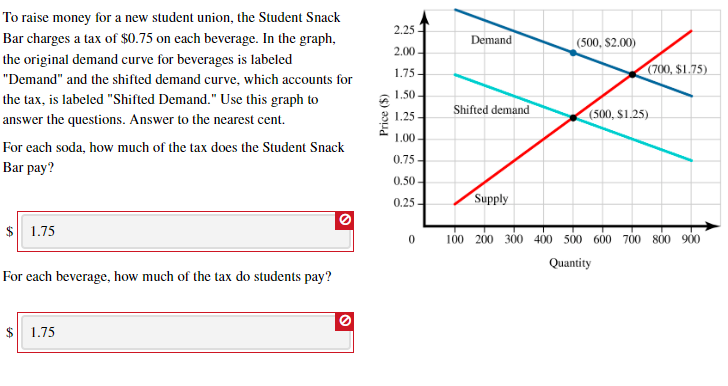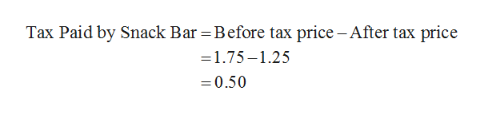# To raise money for a new student union, the Student Snack2.25Bar charges a tax of \$0.75 on each beverage. In the graph,Demand(500, \$2.00)2.00the original demand curve for beverages is labeled(700, \$1.75)1.75"Demand" and the shifted demand curve, which accounts for1.50the tax, is labeled "Shifted Demand." Use this graph toShifted demand(500, S1.25)1.25answer the questions. Answer to the nearest cent.00For each soda, how much of the tax does the Student Snack0.75Bar pay?0.50-Supply0.251.75100 200 300 400 500 600 700 800 900QuantityFor each beverage, how much of the tax do students pay?1.75(\$) aoud

Question
400 viewshelp_outlineImage TranscriptioncloseTo raise money for a new student union, the Student Snack 2.25 Bar charges a tax of \$0.75 on each beverage. In the graph, Demand (500, \$2.00) 2.00 the original demand curve for beverages is labeled (700, \$1.75) 1.75 "Demand" and the shifted demand curve, which accounts for 1.50 the tax, is labeled "Shifted Demand." Use this graph to Shifted demand (500, S1.25) 1.25 answer the questions. Answer to the nearest cent. 00 For each soda, how much of the tax does the Student Snack 0.75 Bar pay? 0.50- Supply 0.25 1.75 100 200 300 400 500 600 700 800 900 Quantity For each beverage, how much of the tax do students pay? 1.75 (\$) aoud fullscreen
check_circle

Step 1

The equilibrium in the market is obtained at the intersection of the demand curve and the supply curve. The demand curve and the supply curve intersected at the point where the price level in the economy is determined to be \$1.75 and the equilibrium quantity demanded and supplied equal to be 700. The imposition of tax caused the demand curve to shift and the new demand curve which shifted downwards intersects with the supply curve at the price point of \$1.25 and the equilibrium quantity of 500.

Step 2

The tax was determined to be \$0.75 which means that the beverages will be supplied to buyers at a price of the determined \$1.25 along with the tax which equals \$2. The amount that the seller receives from the sale of beverages is equal to 1.25 and thus, the tax paid by the student snack bar can be calculated by subtracting the money received by the snack bar after tax from before tax revenue which can be calculated as follows:help_outlineImage TranscriptioncloseTax Paid by Snack Bar = Before tax price - After tax price =1.75-1.25 0.50 fullscreen
Step 3

The above calculation shows that the tax paid by the snack bar is equal to \$0.50 as tax for the student union. Similarly, by subtracting the tax paid by the snack bar from the total per unit t...

### Want to see the full answer?

See Solution

#### Want to see this answer and more?

Solutions are written by subject experts who are available 24/7. Questions are typically answered within 1 hour.*

See Solution
*Response times may vary by subject and question.
Tagged in